Function Repository Resource:

# RatioPartition

Partition a list into sublists with lengths proportional to weights in another list

Contributed by: Daniel Robinson
 ResourceFunction["RatioPartition"][list,{weight1,weight2, …}] splits list into sublists with lengths proportional to weight1,weight2, ….

## Details and Options

ResourceFunction["RatioPartition"][list,{weight1,weight2,},"Randomize"True] randomizes list before splitting into sublists.
The weighti must all be positive real numbers.
Generated sublists always preserve the items in the original list. For this reason, precise partitions are not always possible.

## Examples

### Basic Examples (2)

Partition a list into sublists containing 10%, 30% and 60% of the data:

 In:=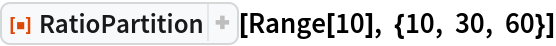Out=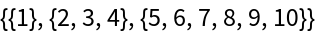Verify that there are no overlapping sublists:

 In:=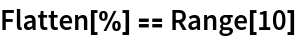Out=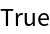Partition a list into sublists containing 22%, 27% and 51% of the data. This time, the partitions can only be approximated:

 In:=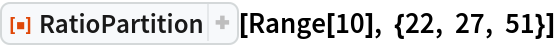Out=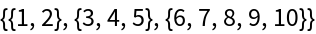### Scope (3)

Partition a list into any number of parts:

 In:=Out=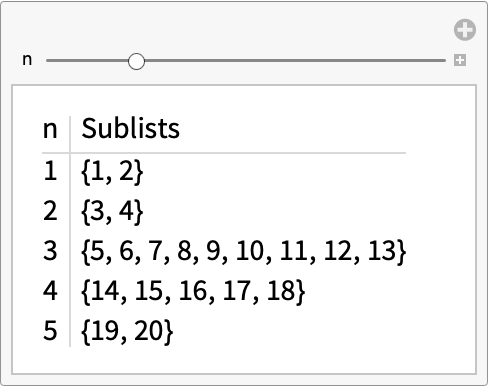Partition a list using decimals or fractions:

 In:=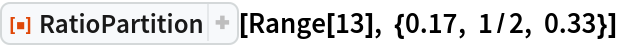Out=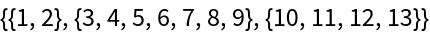Partition a list using weights that don’t sum to a power of 10:

 In:=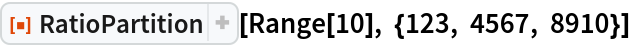Out=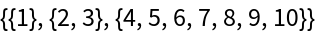### Options (1)

Randomize the data before partitioning with the "Randomize" option:

 In:=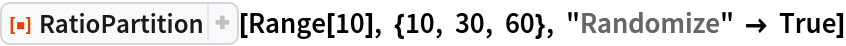Out=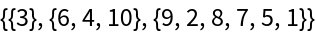### Applications (3)

RatioPartition is useful for separating data for training neural nets. Partition some randomized data into 60% training data, 20% validation data and 20% testing data:

 In:=Train a classifier:

 In:=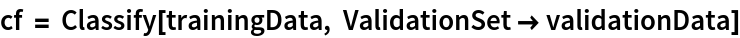Out=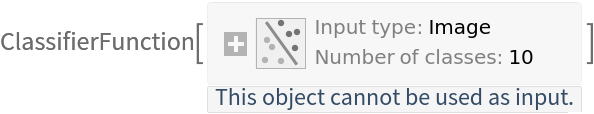Measure the accuracy of the classifier:

 In:=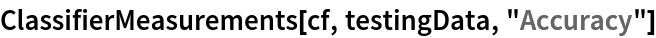Out=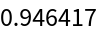### Properties and Relations (3)

When exact partitions are not possible, the order of the weights may affect the lengths of the resulting sublists:

 In:=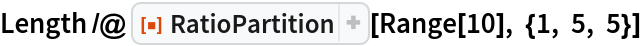Out=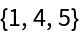In:=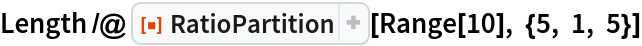Out=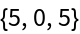The list of weights is scaled to the interval [0, 1]. Therefore, for best results, choose weights that add up to a power of 10. Not doing so may result in sublists of unexpected lengths:

 In:=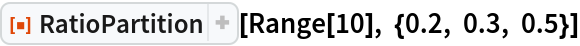Out=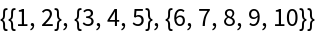In:=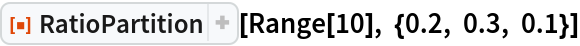Out=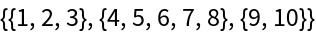Using a single weight will leave the list unchanged (albeit as a sublist):

 In:=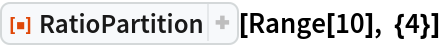Out=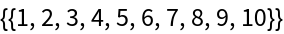### Possible Issues (2)

Due to rounding, it is possible to have empty lists in the output:

 In:=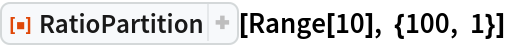Out=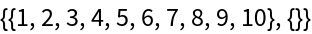Weights must be positive real numbers:

 In:=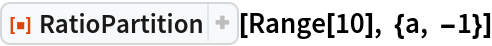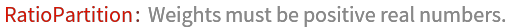Out=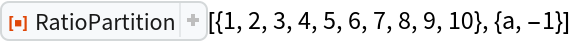Daniel Robinson

## Version History

• 1.0.0 – 27 February 2020

## Author Notes

Future improvements:

Make RatioPartition[list,n] equivalent to Partition[list,n] (while ensuring the "Randomize" option still works).

Make RatioPartition[n] equivalent to RandomPartition[n] (which needs Combinatorica`).